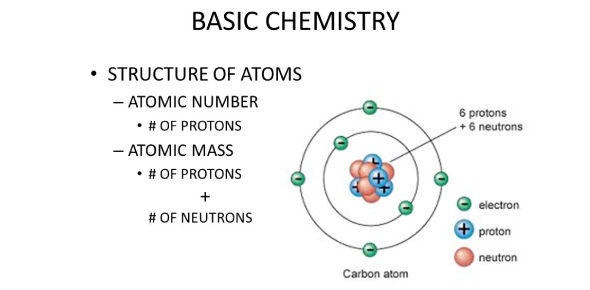# Basic Chemistry Quiz Questions And Answers

10 Questions | Attempts: 18943
ShareSettingsAre you good at chemistry? If yes, then we have a basic chemistry quiz for you. The quiz has very basic chemistry questions to test your knowledge. If you are not clear with your basics, then the quiz might be very tough for you. So, give your best and try to score as much as you can by giving the right answer. Let us go for this quiz now! Good luck!

• 1.
The atom is made of 3 subatomic particles. The subatomic particle found in the nucleus with a positive charge is the ___________.
• A.

Electron

• B.

Neutron

• C.

Proton

• D.

None of these

• 2.
The __________ is the smallest unit of matter.
• A.

Element

• B.

Molecule

• C.

Electron

• D.

Atom

• 3.
In which bond are electrons transferred?
• A.

Covalent

• B.

Ionic

• C.

Double

• D.

None of these

• 4.
_______ has the highest pH value. (Choose the highest one from the options)
• A.

Lime juice

• B.

Caustic soda

• C.

Water

• D.

Wine

• 5.
Carbon's atomic number is
• A.

3

• B.

4

• C.

6

• D.

8

• 6.
An acid has a pH below ______.
• A.

7

• B.

8

• C.

9

• D.

10

• 7.
A base has a pH above _____.
• A.

9

• B.

8

• C.

7

• D.

10

• 8.
Water is made of 1 oxygen molecule, and how many hydrogen atoms?
• A.

3

• B.

2

• C.

1

• D.

5

• 9.
Gold is an example of a ______.
• A.

Molecule

• B.

Element

• C.

Atom

• D.

Covalent bond

• 10.
What is the water molecule if there is an uneven distribution of electrons within the molecule?
• A.

Uneven

• B.

Weird

• C.

Polar

• D.

Strange

## Related TopicsBack to top
×

Wait!
Here's an interesting quiz for you.